# Heart Disease Prediction using ANN

Deep Learning is a technology of which mimics a human brain in the sense that it consists of multiple neurons with multiple layers like a human brain. The network so formed consists of an input layer, an output layer, and one or more hidden layers. The network tries to learn from the data that is fed into it and then performs predictions accordingly. The most basic type of neural network is the ANN (Artificial Neural Network). The ANN does not have any special structure, it just comprises of multiple neural layers to be used for prediction.
Let’s build a model that predicts whether a person has heart disease or not by using ANN.

In the dataset, we have 13 columns in which we are given different attributes such as sex, age, cholesterol level, etc. and we are given a target column which tells us whether that person has heart disease or not. We will keep all the columns as independent variables other than the target column because it will be our dependent variable. We will build an ANN which will predict whether a person has heart disease or not given other attributes of the person.

You can find the dataset here heart disease dataset

Code: Importing Libraries

 `import` `tensorflow as tf ` `import` `pandas as pd ` `import` `numpy as np ` `import` `matplotlib.pyplot as plt ` `import` `keras ` `from` `keras.models ``import` `Sequential ` `from` `keras.layers ``import` `Dense ` `from` `sklearn.metrics ``import` `confusion_matrix `

Code: Importing Dataset

 `data ``=` `pd.read_csv(``'heart.csv'``) ` `data.head() `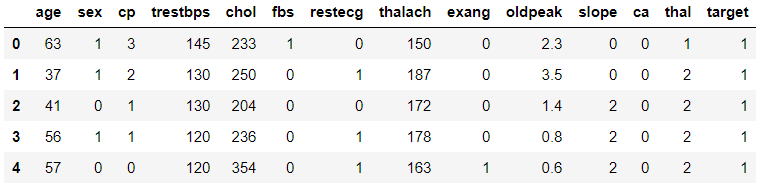Data Description:

 `data.describe() `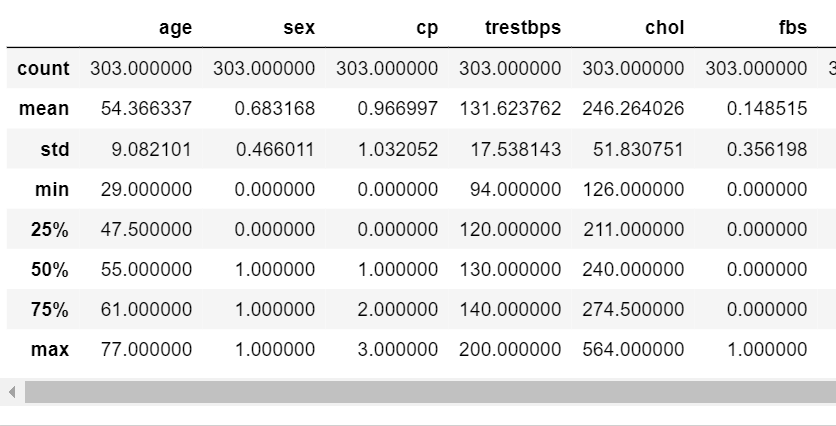Code: Check for null values

 `data.isnull().``any``() `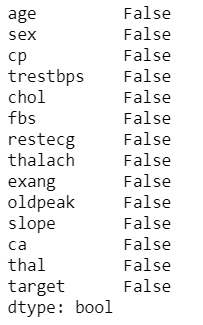Assign Dependent and Independent variable

 `X ``=` `data.iloc[:,:``13``].values ` `y ``=` `data[``"target"``].values `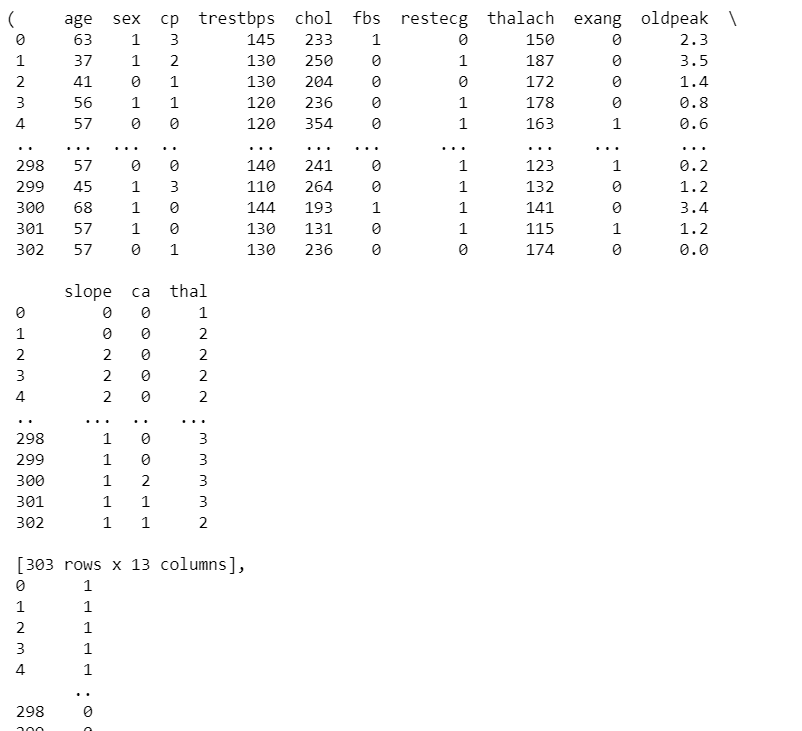Code : Split data into Train and Test dataset

 `X_train,X_test,y_train, y_test ``=` `train_test_split(X,y,test_size ``=` `0.3` `, random_state ``=` `0` `) `

Code: Scale the data.

 `from` `sklearn.preprocessing ``import` `StandardScaler ` `sc ``=` `StandardScaler() ` `X_train ``=` `sc.fit_transform(X_train) ` `X_test ``=` `sc.transform(X_test) `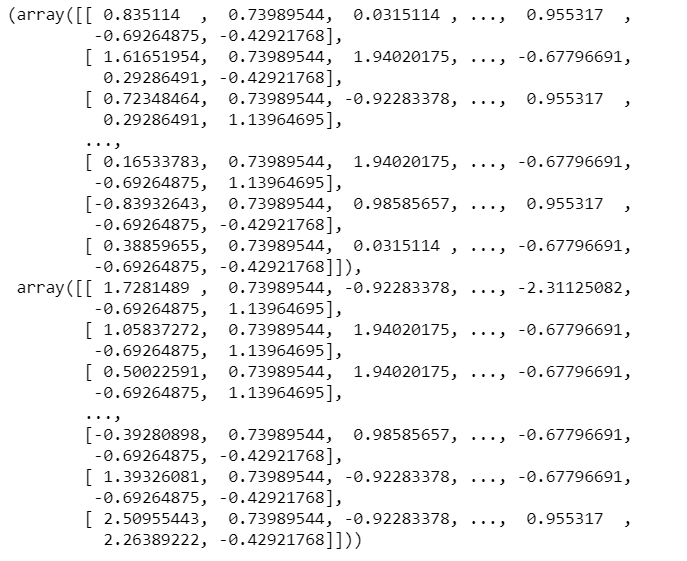Code: Building the Model

 `classifier ``=` `Sequential() ` `classifier.add(Dense(activation ``=` `"relu"``, input_dim ``=` `13``,  ` `                     ``units ``=` `8``, kernel_initializer ``=` `"uniform"``)) ` `classifier.add(Dense(activation ``=` `"relu"``, units ``=` `14``,  ` `                     ``kernel_initializer ``=` `"uniform"``)) ` `classifier.add(Dense(activation ``=` `"sigmoid"``, units ``=` `1``,  ` `                     ``kernel_initializer ``=` `"uniform"``)) ` `classifier.``compile``(optimizer ``=` `'adam'` `, loss ``=` `'binary_crossentropy'``,  ` `                   ``metrics ``=` `[``'accuracy'``] ) `

Code : Fitting the Model

 `classifier.fit(X_train , y_train , batch_size ``=` `8` `,epochs ``=` `100`  `) `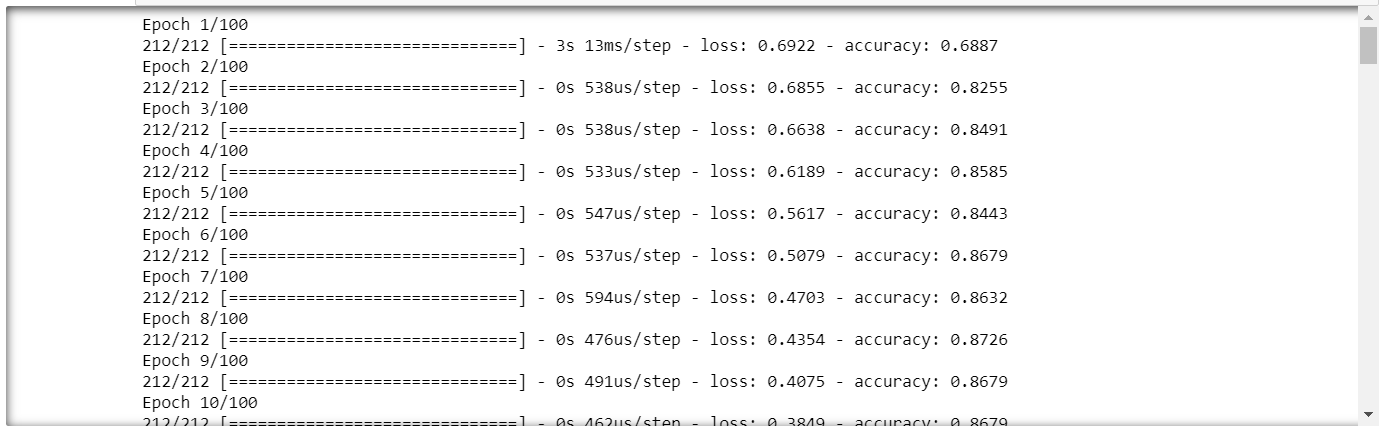Code : Performing prediction and rescaling

 `y_pred ``=` `classifier.predict(X_test) ` `y_pred ``=` `(y_pred > ``0.5``) `

Code: Confusion Matrix

 `cm ``=` `confusion_matrix(y_test,y_pred) ` `cm `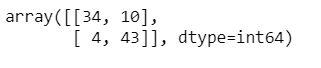Code: Accuracy

 `accuracy ``=` `(cm[``0``][``0``]``+``cm[``1``][``1``])``/``(cm[``0``][``1``] ``+` `cm[``1``][``0``] ``+``cm[``0``][``0``] ``+``cm[``1``][``1``]) ` `print``(accuracy``*``100``) `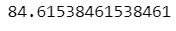We will get accuracy approximately around 85%.

My Personal Notes arrow_drop_upCheck out this Author's contributed articles.

If you like GeeksforGeeks and would like to contribute, you can also write an article using contribute.geeksforgeeks.org or mail your article to contribute@geeksforgeeks.org. See your article appearing on the GeeksforGeeks main page and help other Geeks.

Please Improve this article if you find anything incorrect by clicking on the "Improve Article" button below.

Article Tags :
Practice Tags :

Be the First to upvote.

Please write to us at contribute@geeksforgeeks.org to report any issue with the above content.